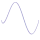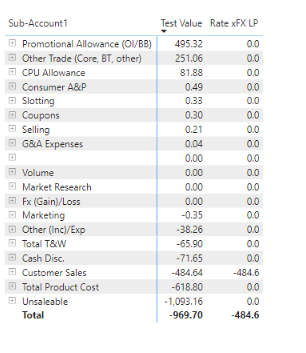cancel
Showing results for
Did you mean:

Fabric is Generally Available. Browse Fabric Presentations. Work towards your Fabric certification with the Cloud Skills Challenge.Frequent Visitor

## Filtering Variable to Return Single Row

Hello All,

I've come across an issue when attempting to set up a measure to be used in a switch function. Below is my current dax expression:

Value =

VAR RatexFX=IF(HASONEFILTER(Account[Sub-Account3]),(Sales/Volume),SUMX(VALUES(Account[Sub-Account3]),(Sales/Volume))-[FX]
RETURN
Calculate(RatexFX,FILTER('Combined P&L','Combined P&L'[Sub-Account3]="Customer Sales"))

In the above measure, I am looking to utilize the RatexFX variable to only show the Rate xFX value for Customer Sales. When done in an individual measure, the desired result is given, but when using Variable, the filter in the Return function does nothing, and the Rate xFX function is shown for all accounts.
9 REPLIES 9Super User

the term "VAR"  is misleading, unfortunately.  It should have been named "CONST"  because that's what it is, a constant.

You need to move it inside the CALCULATE statement to be effective

``````Value =
Calculate(IF(HASONEFILTER(Account[Sub-Account3]),(Sales/Volume),SUMX(VALUES(Account[Sub-Account3]),(Sales/Volume))-[FX],FILTER('Combined P&L','Combined P&L'[Sub-Account3]="Customer Sales"))``````

Might even need to bring the switch out further.Frequent Visitor

Hi Ibendlin,

Appreciate the insight. I provided the above details as a skimmed down version of what I am trying to calculate. In the end DAX expression, I am looking to use a SWITCH formula in a waterfall that then looks at multiple accounts. So in the end it would be:

Value =

VAR RatexFX=IF(HASONEFILTER(Account[Sub-Account3]),(Sales/Volume),SUMX(VALUES(Account[Sub-Account3]),(Sales/Volume))-[FX]
RETURN
Switch(
1, Calculate(RatexFX,FILTER('Combined P&L','Combined P&L'[Sub-Account3]="Customer Sales")),
2, Calculate(RatexFX,FILTER('Combined P&L','Combined P&L'[Sub-Account3]="Marketing Expense")),
3, Calculate(RatexFX,FILTER('Combined P&L','Combined P&L'[Sub-Account3]="Employee Wages")),
4, Calculate(RatexFX,FILTER('Combined P&L','Combined P&L'[Sub-Account3]="SG&A")),
BLANK()).

Thus, the goal of using the variable is to then not have to calculate RatexFX on every step of the SWITCH, but instead perform it once at the beginning. When using the variable, each step of the switch ignores the filter, and instead just displays total Rate xFX.

ThanksSuper User

I already explained why that is the case. You cannot do it this way. Move the SWITCH inside the CALCULATE.Frequent Visitor

Can you show an example of what you mean by inside? Sorry, just a little confused on that.Super User

instead of SWITCH(...,CALCULATE())  you need to do CALCULATE(SWITCH(...))

Please provide sanitized sample data that fully covers your issue. If you paste the data into a table in your post or use one of the file services it will be easier to assist you. I cannot use screenshots of your source data.

Please show the expected outcome based on the sample data you provided. Screenshots of the expected outcome are ok.Frequent Visitor
As a test I have written the below and got the following error:

Test Value =
CALCULATE([Rate xFX],SWITCH(VALUES('NS Account Table'[Index]),
1, FILTER('Combined P&L','Combined P&L'[Sub Account 3]="Customer Sales"),
2, FILTER('Combined P&L','Combined P&L'[Sub Account 3]="CPU Allowance"),
3, FILTER('Combined P&L','Combined P&L'[Sub Account 3]="Slotting"),
4, FILTER('Combined P&L','Combined P&L'[Sub Account 3]="Coupons"),
BLANK()))

A function 'FILTER' has been used in a True/False expression that is used as a table filter expression. This is not allowed.Community Support

Hi @PaulPannu ,

There a syntax error in your formula.

syntax of SWITCH()

SWITCH(<expression>, <value>, <result>[, <value>, <result>]…[, <else>])

You missed <expression> in your code.

Value =

VAR RatexFX=IF(HASONEFILTER(Account[Sub-Account3]),(Sales/Volume),SUMX(VALUES(Account[Sub-Account3]),(Sales/Volume))-[FX]
RETURN
Switch( selectedvalue( waterfall's X-axis ),
1, Calculate(RatexFX,FILTER('Combined P&L','Combined P&L'[Sub-Account3]="Customer Sales")),
2, Calculate(RatexFX,FILTER('Combined P&L','Combined P&L'[Sub-Account3]="Marketing Expense")),
3, Calculate(RatexFX,FILTER('Combined P&L','Combined P&L'[Sub-Account3]="Employee Wages")),
4, Calculate(RatexFX,FILTER('Combined P&L','Combined P&L'[Sub-Account3]="SG&A")),
BLANK()).

If you need more help, please share your example data and expect result.

Best Regards

Community Support Team _ chenwu zhu

If this post helps, then please consider Accept it as the solution to help the other members find it more quickly.Frequent Visitor

Hi, thanks for the tip, but I believe the issue is continuing to persist even with the above solution.

Below you can see the comparison of two DAX expressions:

Test Value =

VAR RatexFX = [Rate xFX]
RETURN
Calculate(RatexFX,FILTER(Account,Account[Sub-Account1]="Customer Sales"))

Rate xFX LP =
CALCULATE (
'Combined P&L'[Rate xFX],
Account[Sub-Account1] = "Customer Sales")
As you can see in the below table, test value continues to do the calculation for all accounts.Community Support

Hi @PaulPannu ,

Measures cannot refer to variables defined outside the measure expression, but can refer to functional scope variables defined within the expression.

VAR keyword (DAX) - DAX | Microsoft Docs

Can you share some example data or pbix file without sensitive data and expect result.

Best Regards

Community Support Team _ chenwu zhu

If this post helps, then please consider Accept it as the solution to help the other members find it more quickly.Announcements#### Power BI Monthly Update - November 2023

Check out the November 2023 Power BI update to learn about new features.#### The largest Power BI and Fabric virtual conference

130+ sessions, 130+ speakers, Product managers, MVPs, and experts. All about Power BI and Fabric. Attend online or watch the recordings.Top Solution Authors
Top Kudoed Authors
Users online (6,531)